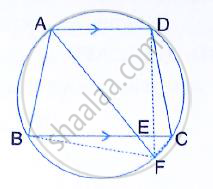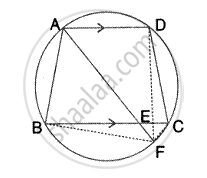Share

In the Following Figure, Abcd is a Cyclic Quadrilateral in Which Ad is Parallel to Bc. If the Bisector of Angle a Meets Bc at Point E and the Given Circle at Point F, Prove That: (I) Ef = Fc (Ii) Bf - ICSE Class 10 - Mathematics

Question

In the following figure, ABCD is a cyclic quadrilateral in which AD is parallel to BC.If the bisector of angle A meets BC at point E and the given circle at point F, Prove that:
(i) EF = FC (ii) BF = DF

SolutionGiven – ABCD is a cyclic quadrilateral in which AD || BC
Bisector of ∠A meets BC at E and the given circle at F. DF and BF are joined.
To prove –
(i) EF = FC
(ii) BF = DF
Proof – ABCD is a cyclic quadrilateral and AD ∥ BC
∵ AF is the bisector of ∠A, ∠BAF = ∠DAF
Also, ∠DAE = ∠BAE

∠DAE = ∠AEB       [Alternate angles]

(i) In ΔABE, ∠ABE = 180° - 2∠AEB

∠CEF = ∠AEB       [vertically opposite angles]

∠ADC = 180° - ABC = 180° - (180° - 2∠AEB)
∠AFC = 180° - ∠ADC

= 180° - 2∠AEB        [since ADFC is a cyclic quadrilateral]

∠ECF = 180° - (∠AFC + ∠CEF)

= 180 - (180 - 2∠AEB + ∠AEB)
= ∠AEB
∴ EC = EF

(ii) ∴ Arc BF = Arc DF      [Equal arcs subtends equal angles]

⇒ BF = DF                      [Equal arcs have equal chords]

Is there an error in this question or solution?
Solution In the Following Figure, Abcd is a Cyclic Quadrilateral in Which Ad is Parallel to Bc. If the Bisector of Angle a Meets Bc at Point E and the Given Circle at Point F, Prove That: (I) Ef = Fc (Ii) Bf Concept: Cyclic Properties.
S